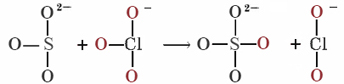# 19.11: Uses of Artificial Isotopes

Tracers

A very large number of isotopes which do not occur naturally can now be made fairly readily by neutron capture using an atomic reactor or a laboratory neutron source. Many of these artificial isotopes have proved very useful in chemistry since they provide a way of identifying atoms from a particular source, a technique known as labeling or tracer study. This technique is particularly easy to use if the isotope is radioactive. Thus, for example, if a small quantity of the radioactive isotope 131I is added in the form of iodide ion to a saturated solution of lead iodide, one soon finds that the solid lead iodide in contact with the solution, as well as the solution, become radioactive. This clearly demonstrates that the solution equilibrium

$\text{PbI}_2 \rightleftharpoons \text{Pb}^{2+} + \text{2I}^{-}$
is a dynamic process involving the constant interchange of iodide ions between the solution and the solid.

Tracer studies are also possible with isotopes which are not radioactive. The isotope 18O is often used in this way, since no convenient radioactive isotope of oxygen is available. In naturally occurring oxygen the isotope 18O is only 0.2 percent of the total. If extra 18O is added, its presence can be detected by mass spectrometry. An interesting and important example of the use of 18O is in the study of photosynthesis. If the water in this reaction is enriched with 18O, then the isotope is found in the oxygen produced:

$6 \text{CO}_{2} (g) + 6 \text{H}_{2} {\text{O}} (l) \rightarrow \text{C}_{6} \text{H}_{12} \text{O}_{6} (s) + 6 {\text{O}}_{2} (g)$ By contrast, if the carbon dioxide is enriched with 18O, none of this enrichment appears in the oxygen produced. Another example of the use of 18O comes from inorganic chemistry. It is the reaction between the sulfite ion and the chlorate ion in aqueous solution:By labeling the oxygens in the chlorate ion, it is found that all the 18O lost from the one species is gained by the other and none of it is transferred to the solvent water. The mechanism of this reaction must thus be a direct transfer of oxygen and is quite unrelated to the two half-equations we conventionally use when balancing the redox equation:

$\text{SO}_{3} \text{ }^{2-} + \text{H}_{2} \text{O} \rightarrow \text{SO}_{4} \text{ }^{2-} + 2\text{H}^{+} + 2e^{-}$
$2 \text{H}^{+} + 2e^{-} + \text{ClO}_{3} \text{ }^{-} \rightarrow \text{ClO}_{2}^{-} + \text{H}_{2} \text{O}$
Neutron Activation Analysis

An important use of radioisotopes in detecting small amounts of certain elements in a sample is neutron activation analysis. The sample being analyzed is irradiated by a neutron source. Nuclei of the element being analyzed capture neutrons, and an unstable nucleus is formed which emits a γ ray. Since the wavelength of this γ ray is characteristic of the element, it can be distinguished from other elements in the sample. This method of analysis has the advantage of being nondestructive. The sample being analyzed is scarcely altered by being irradiated. Neutron activation is also among the most sensitive of analytical techniques. As little as a pictogram (10–12 g) of arsenic, for example, can be detected. This is about 10 000 times more sensitive than Marsh’s test—so often used by the fabled detective Sherlock Holmes. Neutron activation analysis is used by many modern detectives to find evidence of air and water pollution as well as the types of crimes with which Holmes was involved.